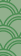Y總，咱們沒有統計pv和uv的系統，預估大約有一千萬uv吧PV是page view的縮寫，即頁面瀏覽量，通常是衡量一個網路新聞頻道或網站甚至一條網路新聞的主要指標。網頁瀏覽數是評價網站流量最常用的指標之一，簡稱為PV

UV是unique visitor的簡寫，是指通過網際網路訪問、瀏覽這個網頁的自然人。pv相比較uv來說，技術上比較容易一些，今天咱們就來說一說uv的統計，為什麼說uv的統計相對來說比較難呢，因為uv涉及到同一個標準下的自然人的去重，尤其是一個uv千萬級別的網站，設計一個好的uv統計系統也許並非想象的那麼容易。IPvarchar(30)客戶端來源ip
DayIDint時間的簡寫，例如 20190629if exists( select 1 from table where ip='ip' and dayid=dayid )
Begin
return 0
End
else
Begin
insert into table .......
End1. 每次請求都需要判斷是否已經存在相同的uv記錄

2. 持久化uv資料不能影響正常的業務

3. uv資料的準確性可以忍受一定程度的誤差class BloomFilter
{
BitArray container = null;
public BloomFilter(int length)
{
container = new BitArray(length);
}

public void Set(string key)
{
var h1 = Hash1(key);
var h2 = Hash2(key);
var h3 = Hash3(key);
var h4 = Hash4(key);
container[h1] = true;
container[h2] = true;
container[h3] = true;
container[h4] = true;

}
public bool Get(string key)
{
var h1 = Hash1(key);
var h2 = Hash2(key);
var h3 = Hash3(key);
var h4 = Hash4(key);

return container[h1] && container[h2] && container[h3] && container[h4];
}

//模擬雜湊函式1
int Hash1(string key)
{
int hash = 5381;
int i;
int count;
char[] bitarray = key.ToCharArray();
count = bitarray.Length;
while (count > 0)
{
hash += (hash << 5) + (bitarray[bitarray.Length - count]);
count--;
}
return (hash & 0x7FFFFFFF) % container.Length;

}
int Hash2(string key)
{
int seed = 131; // 31 131 1313 13131 131313 etc..
int hash = 0;
int count;
char[] bitarray = (key+"key2").ToCharArray();
count = bitarray.Length;
while (count > 0)
{
hash = hash * seed + (bitarray[bitarray.Length - count]);
count--;
}

return (hash & 0x7FFFFFFF)% container.Length;
}
int Hash3(string key)
{
int hash = 0;
int i;
int count;
char[] bitarray = (key + "keykey3").ToCharArray();
count = bitarray.Length;
for (i = 0; i < count; i++)
{
if ((i & 1) == 0)
{
hash ^= ((hash << 7) ^ (bitarray[i]) ^ (hash >> 3));
}
else
{
hash ^= (~((hash << 11) ^ (bitarray[i]) ^ (hash >> 5)));
}
count--;
}

return (hash & 0x7FFFFFFF) % container.Length;

}
int Hash4(string key)
{
int hash = 5381;
int i;
int count;
char[] bitarray = (key + "keykeyke4").ToCharArray();
count = bitarray.Length;
while (count > 0)
{
hash += (hash << 5) + (bitarray[bitarray.Length - count]);
count--;
}
return (hash & 0x7FFFFFFF) % container.Length;
}
}BloomFilter bf = new BloomFilter(200000000);
int exsitNumber = 0;
int noExsitNumber = 0;

for (int i=0;i < 10000000; i++)
{
string key = \$"ip_{i}";
var isExsit= bf.Get(key);
if (isExsit)
{
exsitNumber += 1;
}
else
{
bf.Set(key);
noExsitNumber += 1;
}
}
Console.WriteLine(\$"判斷存在的資料量：{exsitNumber}");
Console.WriteLine(\$"判斷不存在的資料量：{noExsitNumber}");測試結果：